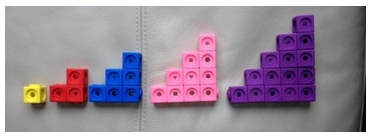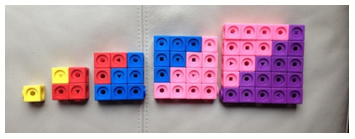# Triangle Numbers in the Classroom

##### Age 11 to 18

Published June 2013.

Triangular numbers are contained in a diverse range of problems and can be linked to a variety of other mathematical topics. We therefore wanted to share our experiences of discovering and using this set of numbers.

Triangular numbers are generally introduced to students using the story of Carl Gauss, a famous mathematician who, as a student, used the idea of the formula for triangular numbers to help him sum consecutive numbers. Clever Carl is an article that describes this story and how it can be applied in different circumstances.

However, triangular numbers actually originate from the Pythagoreans, who developed relationships between geometric shapes and numbers; hence the birth of triangular numbers, square numbers, and pentagonal numbers, etc.

Students can be introduced to triangular numbers using multilink cubes. They could develop the pattern and be able to explain how to get to the next triangle number. From this, students could use the multilink to investigate what happens if you add adjacent triangular numbers.  (These form square numbers as pictured.)Another NRICH task which links triangle numbers to multiplication is Triangle Numbers, where students develop patterns in triangle numbers on a multiplication square. This task can be used to start to visualize a pattern in the sequence of triangular numbers.

An investigation which provides a nice icebreaker with a group of students is ‘How many names?’.
The example described below was conducted by the PGCE students of 2013 on their first day at the University of Cambridge.

The group consisted of 21 people split into 10 groups. 9 of these groups were pairs and there was one group of three. The groups were given a few minutes to get to know each other. Then the first pair introduced each other and everyone in the group said the names of those two people.
Next, the second pair introduced each other and everyone in the group said the names of all four of the people who had been introduced so far.  After that we said six names (including the two people introduced in the third group), and so on.  How many names were spoken altogether after everyone had been introduced and named?
It is easy for us to work out the number of groups that were said, because this is a triangle number (the 10th one: ${\bf \frac{10x11}{2}} = 55$).
If every group included two people then we would need to double this number to get 110 names. Since one group had three members the number of names to add depends on their position within the circle.  They were in group 8, so this led to the extra person's name being said in the 8th, 9th and 10th rounds, which is 3 times. The total number of names that were said is therefore 113.

What would have happened if this task was carried out by a tutor group of 30 pupils?  What if one of the pupils was away?  Or what if the tutor joined in too?  There are lots of ways that this problem can be extended!

Other contextual investigations that also involve triangle numbers in their solution are Mystic Rose and Handshakes.
When we have used these investigations, we have found that students are very good at examining the problem and developing some very interesting and individual methods of finding solutions.

We have found that students are able to recognise after 4 or 5 terms that the answer can be found by adding consecutive terms. For example:  to find the 5th term you can either work out 1+2+3+4+5, or do 10 (the previous term) + 5. Many students struggle, however, when they try to go from this to the general formula for triangle numbers of

${\bf \frac{n(n+1)}{2}}$

A task that may be useful to make the link between these two ideas is Picturing Triangle Numbers.

This formula can then be used to discuss: sequences, specifically quadratic sequences; the nth term and substitution into algebraic expressions.

Route to Infinity is a slightly more challenging problem, with its basic result forming the triangle number sequence. However, route to infinity can be extended beyond the triangle numbers by examining routes to specific co-ordinates, which requires visualization and producing a systematic approach to reach a suitable conclusion. A complex understanding of the formula could be necessary for some of the questions in the problem and it could be further extended to look at infinity and countable infinities.

A final application of triangle numbers that we discovered was listing outcomes through sample space diagrams. If two independent but identical events were to occur, a sample space diagram would often be used to display all of the possible outcomes. If you were then to ask students to find the number of different outcomes there were to the problem and it was such that the order of events was not important, students would find that their answer was a triangle number. If the repeated events were dismissed in a systematic way, an obvious triangle in one of the corners would be seen, reinforcing this to students.

We have found during the course of the year that triangle numbers appear in many different problems and have realized that triangle numbers are an important part of a mathematician’s toolkit.